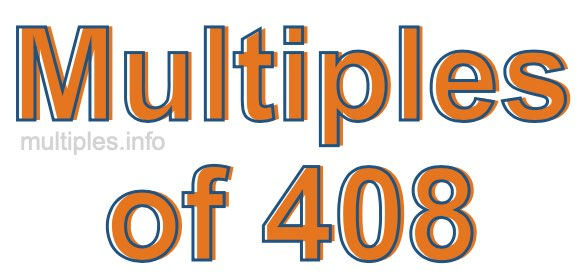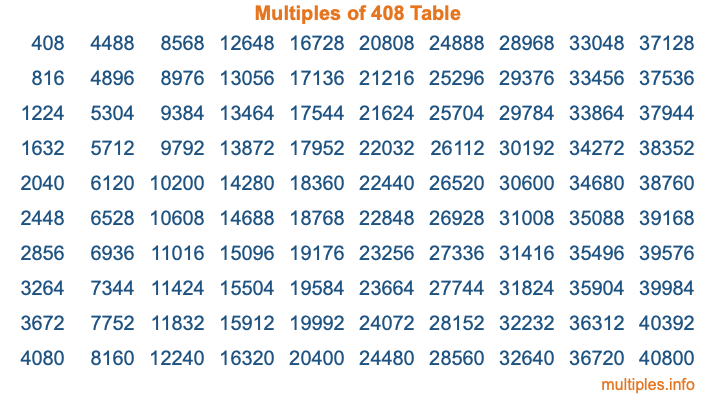Multiples of 408Welcome to the Multiples of 408 page. Here we will first teach you everything you will ever need to know about the multiples of 408, and then give you a study guide summary of everything we taught you to make sure you remember it all. Use this page to look up facts and learn information about the multiples of 408. This page will make you a multiples of four hundred eight expert!

Definition of Multiples of 408
Multiples of 408 are all the numbers that when divided by 408 equal an integer. Each of the multiples of 408 are called a multiple. A multiple of 408 is created by multiplying 408 by an integer.

Therefore, to create a list of multiples of 408, you start with 1 multiplied by 408, then 2 multiplied by 408, then 3 multiplied by 408, and so on for as long as you want. Thus, the list of the first five multiples of 408 is 408, 816, 1224, 1632, and 2040. To see a larger list of multiples of 408, see the printable image of Multiples of 408 further down on this page. We also have a category where you can choose any nth multiple of 408.

Multiples of 408 Checker
The Multiples of 408 Checker below checks to see if any number of your choice is a multiple of 408. In other words, it checks to see if there is any number (integer) that when multiplied by 408 will equal your number. To do that, we divide your number by 408. If the the quotient is an integer, then your number is a multiple of 408.

Is  a multiple of 408?

Least Common Multiple of 408 and ...
A Least Common Multiple (LCM) is the lowest multiple that two or more numbers have in common. This is also called the smallest common multiple or lowest common multiple and is useful to know when you are adding our subtracting fractions. Enter one or more numbers below (408 is already entered) to find the LCM.

Check out our LCM Calculator if you need more details about the Least Common Multiple or if you need the LCM for different numbers for adding and subtraction fractions.

nth Multiple of 408
As we stated above, 408 is the first multiple of 408, 816 is the second multiple of 408, 1224 is the third multiple of 408, and so on. Enter a number below to find the nth multiple of 408.

th multiple of 408

Multiples of 408 vs Factors of 408
408 is a multiple of 408 and a factor of 408, but that is where the similarities end. All postive multiples of 408 are 408 or greater than 408. All positive factors of 408 are 408 or less than 408.

Below is the beginning list of multiples of 408 and the factors of 408 so you can compare:

Multiples of 408: 408, 816, 1224, 1632, 2040, etc.

Factors of 408: 1, 2, 3, 4, 6, 8, 12, 17, 24, 34, 51, 68, 102, 136, 204, 408

As you can see, the multiples of 408 are all the numbers that you can divide by 408 to get a whole number. The factors of 408, on the other hand, are all the whole numbers that you can multiply by another whole number to get 408.

It's also interesting to note that if a number (x) is a factor of 408, then 408 will also be a multiple of that number (x).

Multiples of 408 vs Divisors of 408
The divisors of 408 are all the integers that 408 can be divided by evenly. Below is a list of the divisors of 408.

Divisors of 408: 1, 2, 3, 4, 6, 8, 12, 17, 24, 34, 51, 68, 102, 136, 204, 408

The interesting thing to note here is that if you take any multiple of 408 and divide it by a divisor of 408, you will see that the quotient is an integer.

Multiples of 408 Table
Below is an image of the first 100 multiples of 408 in a table. The table is in chronological order, column by column. The first column has the first ten multiples of 408, the second column has the next ten multiples of 408, and so on.The Multiples of 408 Table is also referred to as the 408 Times Table or Times Table of 408. You are welcome to print out our table for your studies.

Negative Multiples of 408
Although not often discussed or needed in math, it is worth mentioning that you can make a list of negative multiples of 408 by multiplying 408 by -1, then by -2, then by -3, and so on, to get the following list of negative multiples of 408:

-408, -816, -1224, -1632, -2040, etc.

Multiples of 408 Summary
Below is a summary of important Multiples of 408 facts that we have discussed on this page. To retain the knowledge on this page, we recommend that you read through the summary and explain to yourself or a study partner why they hold true.

There are an infinite number of multiples of 408.

A multiple of 408 divided by 408 will equal a whole number.

408 divided by a factor of 408 equals a divisor of 408.

The nth multiple of 408 is n times 408.

The largest factor of 408 is equal to the first positive multiple of 408.

408 is a multiple of every factor of 408.

408 is a multiple of 408.

A multiple of 408 divided by a divisor of 408 equals an integer.

408 divided by a divisor of 408 equals a factor of 408.

Any integer times 408 will equal a multiple of 408.

Multiples of a Number
Here you can get the multiples of another number, all with the same attention to detail as we did for multiples of 408 on this page.

Multiples of
Multiples of 409
Did you find our page about multiples of four hundred eight educational? Do you want more knowledge? Check out the multiples of the next number on our list!